Here is the way to install packages, you need to install them only once.

install.packages(c("ade4","FactoMiner","factoextra", "ggplot2", "tidyverse", "ggpubr"))

There is other way to install some packages, indeed some packages are not on the R repositery name CRAN.
They can be stored in GitHub or on bioconductor.
WE WILL SEE THAT LATER

#you need to lauch the packages each time you open a R session
library(FactoMineR)
library(factoextra)
library(ggplot2)
library(tidyverse)
library(ggpubr)

### Work in a directory

As you created a project your directory will be the one from your project, everything you will create and save will be store in this file

getwd()#will give you the present directory
setwd(dirname(file.choose()))#will open a browser to choose the directory
setwd(dir = "/home/remy/Documents/Vidéo R/xaringan-themer.css") #set it by typing or pasting from your computer

### First task : import your dataset

Package comes with stored dataset that you can call easily by using data() function.

#Import data from a package
data(iris) #a common set used in most tutorial on the net, its from ggplot2

And you can import your own data. It is recommended to import them by typing, instead of enter them by the “Import Dataset” button. To prevent error when typing your file path, go to your file, right-click on it and copy the path of your file, then paste it on R.

#Import your own data
getwd()
##  "/home/remy/Documents/Vidéo R"
csv <- read.csv(file = "Acides_biliaires_feces_practice_R.csv", sep=",")
rownames(csv) <- csv$Mice_number Or you can launch a browser using this function : file.choose() csv<- read.csv(file.choose(), sep = ",") #will open a browser where you can select the file you want For excel files you need to use the package readxl, don’t forget to specify the sheet you want to use library(readxl) excel<- read_excel(file.choose(), sheet = "Feuil1") Now that you stored your data in the environment, you can see it either by clicking on it or by : View(csv) You can also type the name of your object, here csv, and press CTRL+ENTER and it will be print the console head(csv,5) ### Now you can discuss with your data. There is two way to dialog with your data :  #The dollar sign allows you to call for columns (doesn't work for rows) csv$
#The backets allows you access both rows and columns, before the comma you have the rows, after you have the columns
csv[,]

Example of various ways to call on your data

csv$Treatment ; csv[,2] ; csv[,"Treatment"]; #these two ways are equal ##  "Cefotaxime_Neomycin" "Cefotaxime_Neomycin" "Cefotaxime_Neomycin" ##  "Cefotaxime_Neomycin" "Vancomycin_Imipenem" "Vancomycin_Imipenem" ##  "Vancomycin_Imipenem" "Vancomycin_Imipenem" "Vancomycin_Imipenem" ##  "Vancomycin_Imipenem" "Control" "Control" ##  "Control" "Control" "Cefotaxime_Neomycin" ##  "Control" ##  "Cefotaxime_Neomycin" "Cefotaxime_Neomycin" "Cefotaxime_Neomycin" ##  "Cefotaxime_Neomycin" "Vancomycin_Imipenem" "Vancomycin_Imipenem" ##  "Vancomycin_Imipenem" "Vancomycin_Imipenem" "Vancomycin_Imipenem" ##  "Vancomycin_Imipenem" "Control" "Control" ##  "Control" "Control" "Cefotaxime_Neomycin" ##  "Control" ##  "Cefotaxime_Neomycin" "Cefotaxime_Neomycin" "Cefotaxime_Neomycin" ##  "Cefotaxime_Neomycin" "Vancomycin_Imipenem" "Vancomycin_Imipenem" ##  "Vancomycin_Imipenem" "Vancomycin_Imipenem" "Vancomycin_Imipenem" ##  "Vancomycin_Imipenem" "Control" "Control" ##  "Control" "Control" "Cefotaxime_Neomycin" ##  "Control" csv[2,] ; csv["F-2",]#here to # Use functions from package ### Make simple task R allows us to perform multiple task : - Stats - Algorythm - Plots (coming after) We will start by making some stats. Does my data follow a normal distribution ? For that purpose we will use the Shapiro test : shapiro.test(csv$TUDCA)
##
##  Shapiro-Wilk normality test
##
## data:  csv$TUDCA ## W = 0.96686, p-value = 0.7856 hist(csv$TUDCA)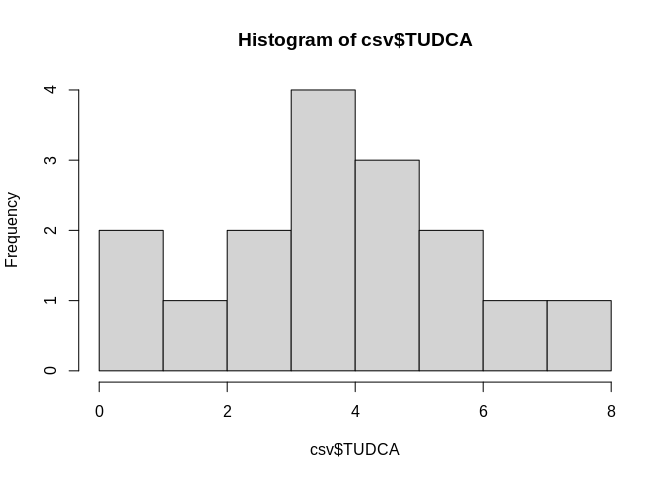plot(csv$TUDCA)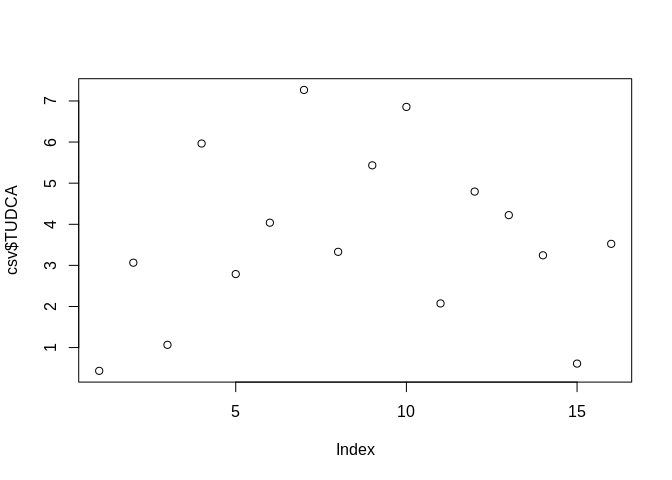The data is not different from the normality so we can use a parametric test : an Anova test with the aov function. x <- aov(formula= TUDCA~Treatment, csv) summary(x) ## Df Sum Sq Mean Sq F value Pr(>F) ## Treatment 2 20.30 10.151 3.036 0.0828 . ## Residuals 13 43.47 3.344 ## --- ## Signif. codes: 0 '***' 0.001 '**' 0.01 '*' 0.05 '.' 0.1 ' ' 1 Seems that this bile acid is different between groups. If the dataset didn’t follow a Normal distribution we could have used the Kruskal-Wallis test. kruskal.test(TUDCA~Treatment, csv) Just to be sure we will perform a Student test two by two by subseting the dataset. We will use the subset() function. Ctrl_CN <- subset(csv, subset = Treatment!="Vancomycin_Imipenem" ) Ctrl_VI <- subset(csv, subset = Treatment!="Cefotaxime_Neomycin" ) t.test(TUDCA~Treatment, Ctrl_CN) ; t.test(TUDCA~Treatment, Ctrl_VI) ## ## Welch Two Sample t-test ## ## data: TUDCA by Treatment ## t = -1.1761, df = 5.5069, p-value = 0.2879 ## alternative hypothesis: true difference in means between group Cefotaxime_Neomycin and group Control is not equal to 0 ## 95 percent confidence interval: ## -4.201347 1.513859 ## sample estimates: ## mean in group Cefotaxime_Neomycin mean in group Control ## 2.228228 3.571972 ## ## Welch Two Sample t-test ## ## data: TUDCA by Treatment ## t = -1.5504, df = 8.0079, p-value = 0.1596 ## alternative hypothesis: true difference in means between group Control and group Vancomycin_Imipenem is not equal to 0 ## 95 percent confidence interval: ## -3.4321582 0.6721982 ## sample estimates: ## mean in group Control mean in group Vancomycin_Imipenem ## 3.571972 4.951952 There is no difference for this bile acid. ### Let’s make our first plot. Here we want to use the base function form R. R comes with a base structure with a lot of function. Here we’re gonna plot the Treatment column with the TUDCA using the plot() function. Here we’re gonna make a boxplot and add colors based on the treatment groups boxplot(TUDCA~Treatment, data= csv, col= c("red","black", "orange"))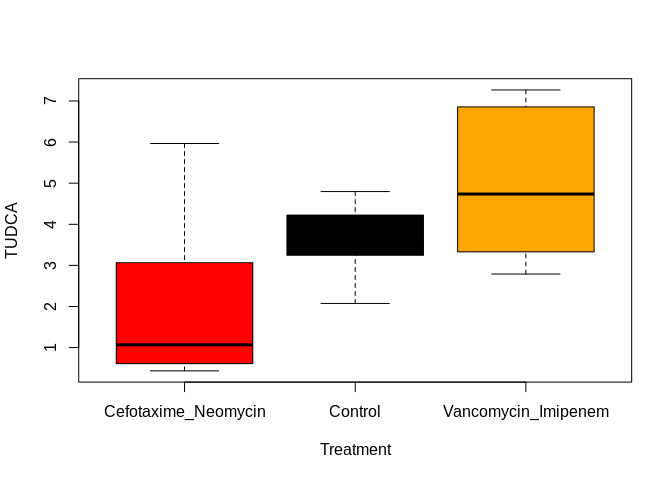The same plot but with a package name ggplot2 library(ggplot2) p1<- ggplot(csv, aes(x = Treatment, y= TUDCA, fill=Treatment))+geom_boxplot()#We use the geom_boxplot to make box plot p2<- ggplot(csv, aes(x = Treatment, y= TUDCA, fill=Treatment))+geom_col()#As column plot p3<- ggplot(csv, aes(x = Treatment, y= TUDCA, fill=Treatment))+geom_violin()#As violin plot p4 <-ggplot(csv, aes(x = Treatment, y= TUDCA, color=Treatment))+geom_point()#As plot point library(ggpubr) #to arrange the plots in the same grid ggarrange(p1, p2, p3, p4)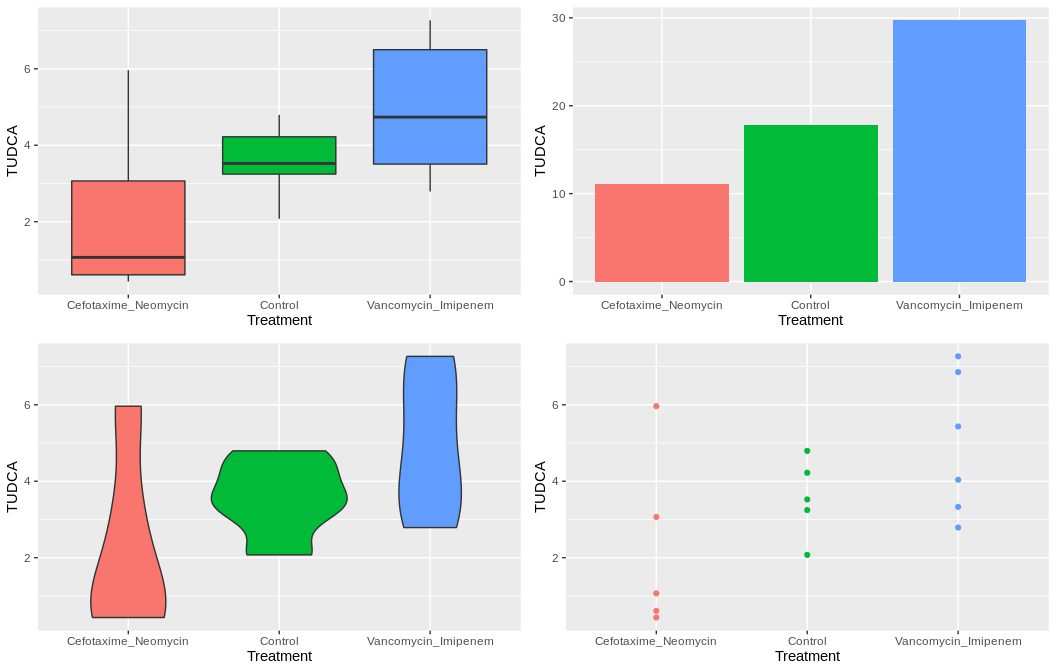There is a multitude of ways to modify the plots. First, we will change the global theme of the plots by using theme_set(). #These are some of the theme you can use p <- ggplot(csv, aes(x = Treatment, y= TUDCA, fill=Treatment))+geom_boxplot() class <- p+ theme_classic()+theme(legend.position = "none") #remove legend bw <- p+ theme_bw()+theme(legend.position = "none") #remove legend void<- p+ theme_void()+theme(legend.position = "none") #remove legend mini <- p+ theme_minimal()+theme(legend.position = "none") #remove legend ggarrange(class, bw, void, mini)+theme(legend.position = "none") #remove legend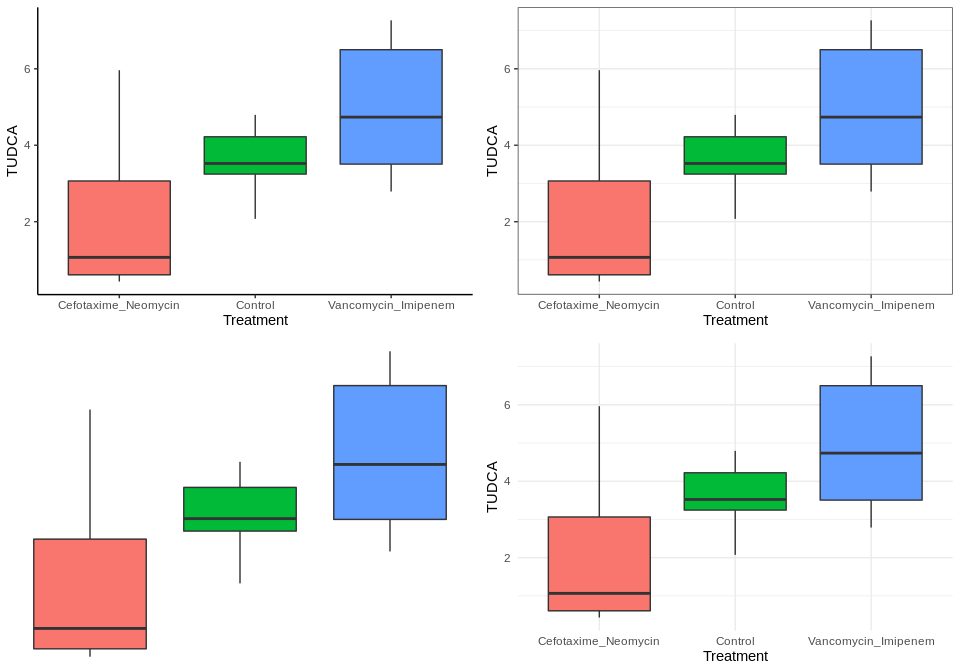In addition to premade theme, you can modify any aspect of the plot easily by using theme() function. All the modifications will fall under 4 types of element : - element_text: to modify text appearance - element_line: to modify line appearance - element_rect: to modify rectangle appareance - element_blank: to make the element blank #We want here to remove the title from the x axis and the legend : ggplot(csv, aes(x = Treatment, y= TUDCA, fill=Treatment))+geom_boxplot()+ theme(axis.title.x = element_blank(), legend.position = "none")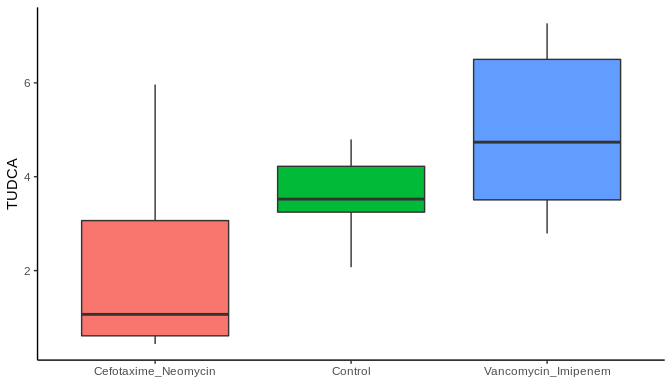# Here we want to make the text from x axis with an angle of 30 degres and make the y axis line as a dashed line ggplot(csv, aes(x = Treatment, y= TUDCA, fill=Treatment))+geom_boxplot()+ theme(axis.title.x = element_blank(), legend.position = "none", axis.text.x = element_text(angle = 30), axis.line.y = element_line(linetype = "dashed"))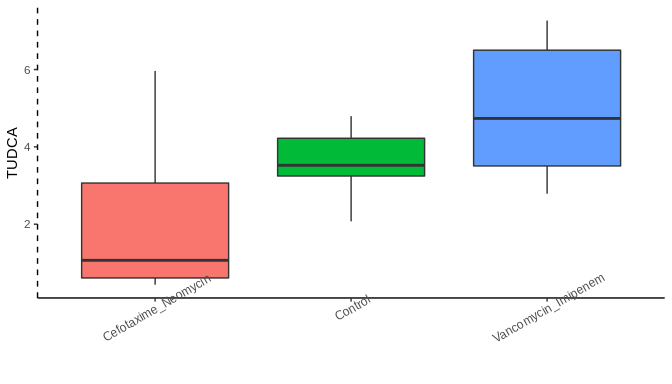You can now play with theme from ggplot and modify as you want your plots. We now want to modify the colors. For that we’re gonna use argument scale_ … This is an argument which allows you to modify a lot of element from the plot. You can modify the colors, the breaks, etc. ggplot(csv, aes(x = Treatment, y= TUDCA, fill=Treatment))+geom_boxplot()+ theme(axis.title.x = element_blank(), legend.position = "none")+scale_fill_manual(values = c("brown","orange","pink"))#you can set manually colors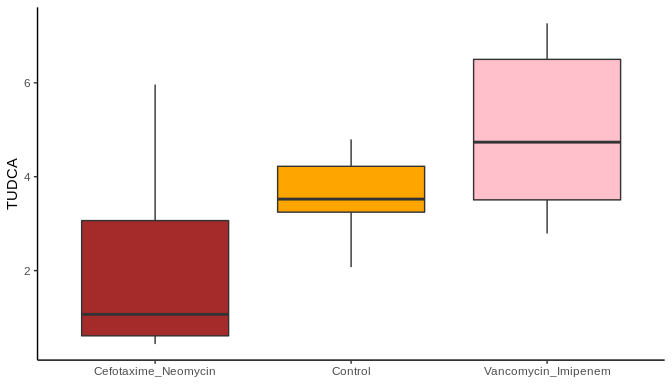library(ggsci) ggplot(csv, aes(x = Treatment, y= TUDCA, fill=Treatment))+geom_boxplot()+ theme(axis.title.x = element_blank(), legend.position = "none")+scale_fill_futurama("planetexpress")#or use a predefined palette from a package, here we use the package ggsci and it futurama palette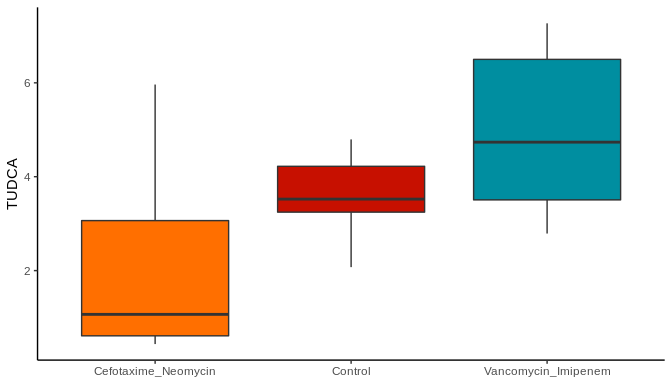Finally, you can add multiple geom to your plots. For example we want here to put some jitters on our boxplot to see the distribution of the data. To make nicer I will reduce the opacity of my boxplots by using alpha= and add color to my jitters by using color= Treatment. ggplot(csv, aes(x = Treatment, y= TDCA))+ geom_boxplot(alpha=0.3, aes(fill=Treatment))+ geom_jitter(aes(color=Treatment))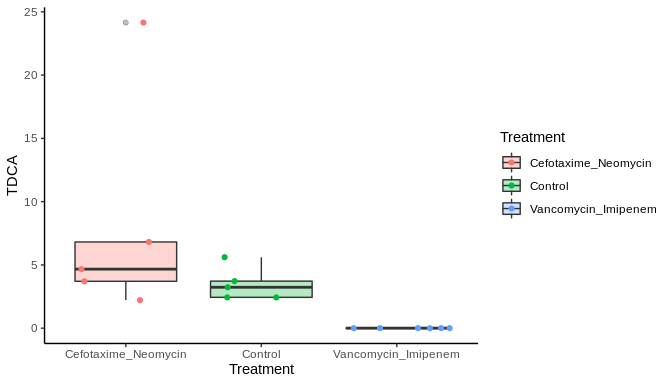## Make some modifications on our data As we can see on the dataset, we have a lot of variables and we don’t want to repeat the plots by changing one by one the variables. We also want in this case to make a plot with all the variables as stacked barplot. For that purpose we will use the tidyr package, which allows us to perform essential modifications on our dataset. For now our dataset is on a large format and we want him on a long format. Here is how we proceed : library(tidyr) csv_long <- gather(csv, key= "Bile_acids", value = "Value", -Treatment, -Mice_number)#We are creating a new column with all bile acids name and another one which contains the values for each dim(csv) ##  16 32 dim(csv_long) ##  480 4 head(csv_long, 20) We can now plot all bile acids as one ! We change the parameters to Value for y axis, we want to color by bile acid belonging and add the geom_bar(position=“fill”) to make a stacked barplot. Of note, you can try to use position=“stack” and see what happens. ggplot(csv_long, aes(x= Treatment, y= Value, fill=Bile_acids))+geom_bar(stat="identity", position = "fill")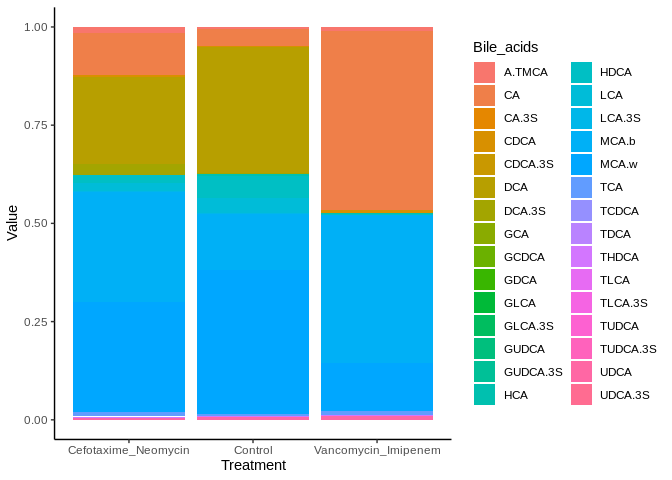We took a large dataset and transformed it to a long one. We can make the contrary as above. csv_large <- spread(csv_long, key=Bile_acids, value= Value) dim(csv_large) ##  16 32 head(csv_large, 5) #we have now the same format as the beginning ## Let’s get a little deeper in data mining. One problem when we want to show our data is the succession of boxplot or dotplot with a different condition or a different marker. Here we have 32 bile acids, we are not gonna show 32 different plot, we are gonna use multiparametric analysis or heatmaps. The easiest way to perform a multiparametric analysis is to use the PCA (Principal Composant Analysis) : #PCA doesn't like 0, so we will remove them first no_zero_dans_ma_team= csv%>% select(where(~any(. != 0))) myfirstpca= PCA(no_zero_dans_ma_team, scale.unit = T, quali.sup = 1:2 )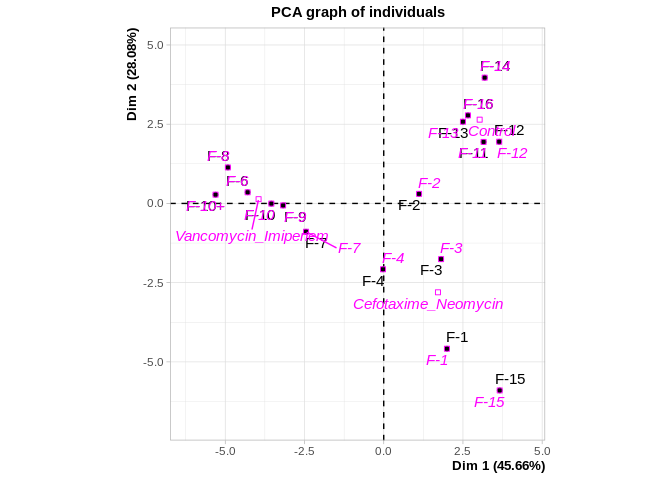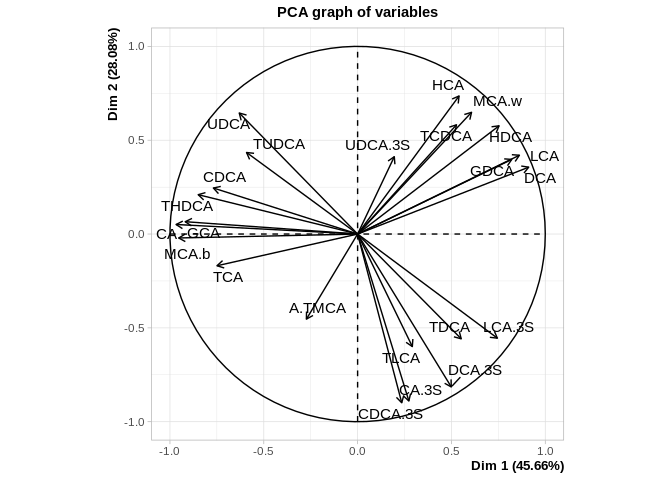We have our first PCA ever ! Good job. Now let’s try to make it a little nicer. We will use the package factorextra to ameliorate our PCA. Let’s try to visualize the PCA for the individuals, the mice. Let’s start with the most basic plot : #We want to use our treatment group info and also the names of the mice grp = as.factor(no_zero_dans_ma_team$Treatment)
ind= as.factor(no_zero_dans_ma_team$Mice_number) fviz_pca_ind(myfirstpca)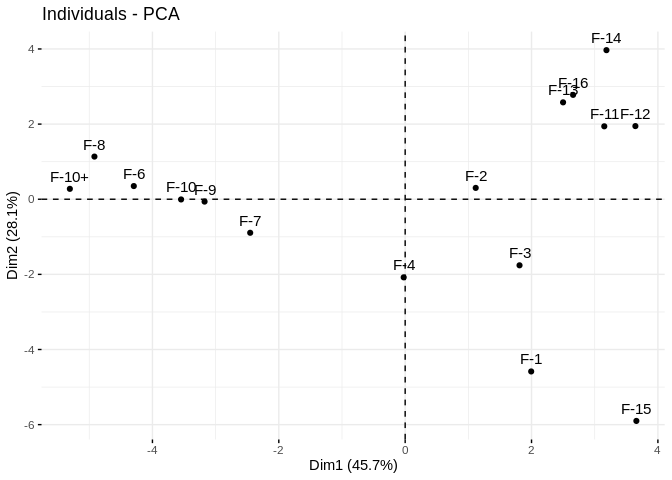Now let’s add the group names and some colors fviz_pca_ind(myfirstpca, col.ind = grp)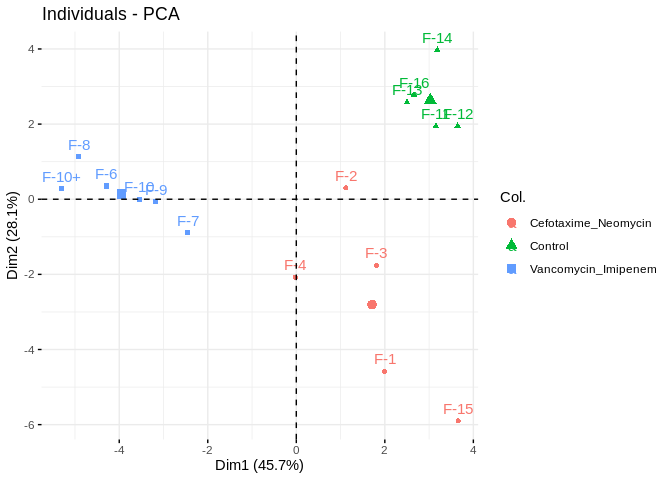And ellipses around the groups: fviz_pca_ind(myfirstpca, col.ind = grp, addEllipses = T)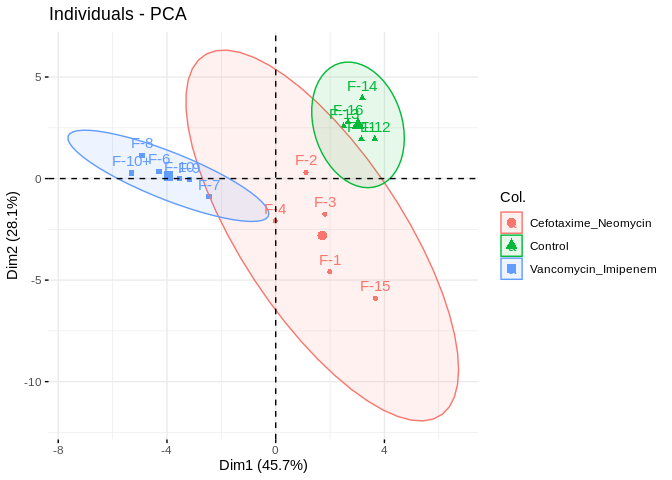Change the colors: fviz_pca_ind(myfirstpca, col.ind = grp, addEllipses = T, palette = c("orange", "darkblue","darkgreen"), repel = T)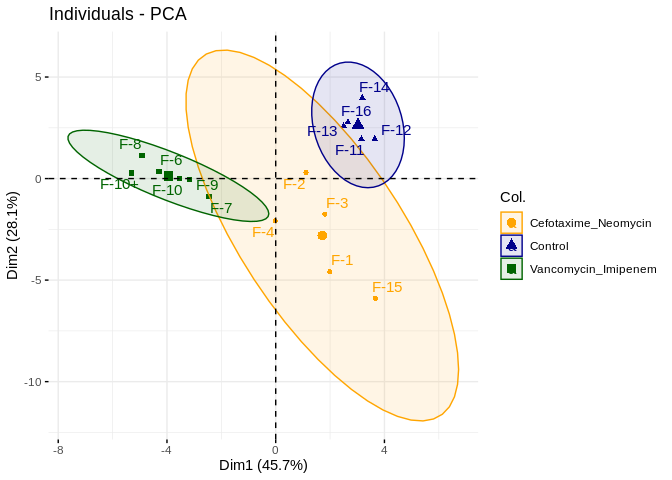You can change a multitude of settings to make your plot look prettier. You can also make fviz_pca_biplot or more depending on what you want to visualize. Here is a nice tutorial focused on PCA: http://www.sthda.com/french/articles/38-methodes-des-composantes-principales-dans-r-guide-pratique/73-acp-analyse-en-composantes-principales-avec-r-l-essentiel/ I prefer the ade4 package. It’s a little bit harder to use but gives very nice plots. ade4 is based on R base package and plotting device on the contrary factoextra is based on ggplot2. The first step is to create a dudi object which will be use to plot afterwards. ade4 won’t accept the pca object from FactoMiner so you need to use dudi.pca, however, factoextra accepts dudi objects. The package will ask you the number of axis to keep, you can skip this step by adding : , scannf = F, nf=2. # Putting this here no_zero_dans_ma_team= csv%>% select(where(~any(. != 0))) grp = as.factor(no_zero_dans_ma_team$Treatment)
ind= as.factor(no_zero_dans_ma_team$Mice_number) mysecondpca = dudi.pca(no_zero_dans_ma_team[, -c(1:2)], center = T, scale=T, scannf = F, nf=2) We can now plot the PCA. For that purpose we have a lot of choice but I personally like to plot the PCA with ellipses based on the groups using s.class(). The dudi object contains multiple class in a list format, we need for this specific function to use$l1 and give the grp factor we created before.

par(mfrow=c(2,2))
s.class(mysecondpca$l1, fac = grp, col=c("orange", "darkblue","darkgreen"))# give it somme colors s.class(mysecondpca$l1, fac = grp, col=c("orange", "darkblue","darkgreen"), grid = F)# remove the grid
s.class(mysecondpca$l1, fac = grp, col=c("orange", "darkblue","darkgreen"), grid = F, clabel = 2)# change the size of the text s.class(mysecondpca$l1, fac = grp, col=c("orange", "darkblue","darkgreen"), grid = F, clabel = 2, cpoint = 2) #change the point size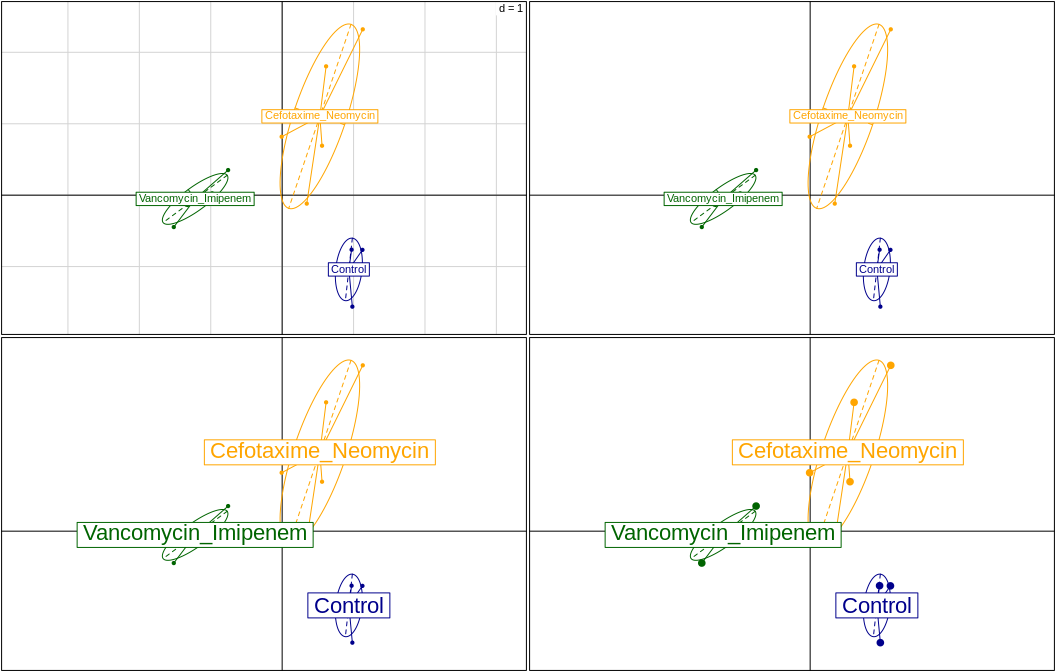## What about heatmaps ?

Heatmap is very powerfull way of plotting multiparametric data and really easy to read when you know what to look. This a really better way to plot your data than making a multitude of plots